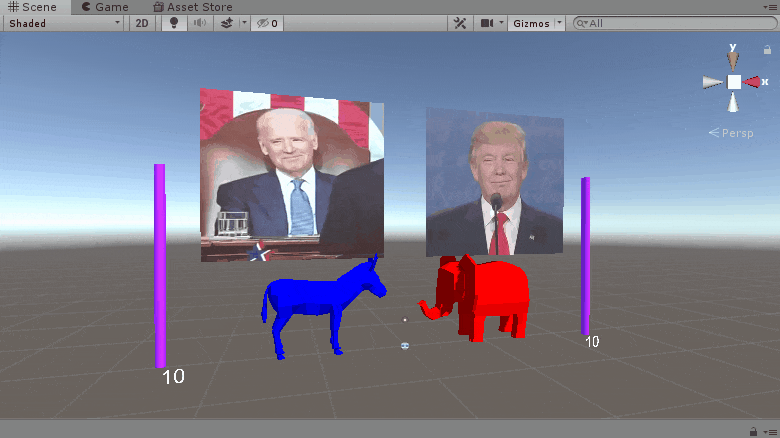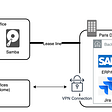# Longest Common Subsequence (Top-Down)— Day 52(Python)

Today’s article is the second part of the three-part series of Longest Common Subsequence(LCS) problem. You can follow this link if you need to understand how to solve the LCS problem using recursion.

1143. Longest Common Subsequence

Given two strings `text1` and `text2`, return the length of their longest common subsequence.

A subsequence of a string is a new string generated from the original string with some characters(can be none) deleted without changing the relative order of the remaining characters. (eg, “ace” is a subsequence of “abcde” while “aec” is not). A common subsequence of two strings is a subsequence that is common to both strings.

If there is no common subsequence, return 0.

Example 1:

`Input: text1 = "abcde", text2 = "ace" Output: 3  Explanation: The longest common subsequence is "ace" and its length is 3.`

Example 2:

`Input: text1 = "abc", text2 = "abc"Output: 3Explanation: The longest common subsequence is "abc" and its length is 3.`

Example 3:

`Input: text1 = "abc", text2 = "def"Output: 0Explanation: There is no such common subsequence, so the result is 0.`

Constraints:

• `1 <= text1.length <= 1000`
• `1 <= text2.length <= 1000`
• The input strings consist of lowercase English characters only.

Before we learn about Top-Down method, let us recall the code for LCS using recursion.

`class FindLongest:    def longestCommonSubsequence(self, text1: str, text2: str) -> int:        if len(text1) == 0 or len(text2) == 0:            return 0        if text1[-1] == text2[-1]:            return 1+(self.longestCommonSubsequence(text1[:-1],text2[:-1]))        else:            return max(self.longestCommonSubsequence(text1[:-1],text2), self.longestCommonSubsequence(text1,text2[:-1]))`

In the above code, we are using recursion to find the length of longest common subsequence. If we observe the last line, we see a presence of overlapping subproblem.

`return max(self.longestCommonSubsequence(text1[:-1],text2), self.longestCommonSubsequence(text1,text2[:-1]))`

Confused? Let us take an example.

`Input:text1 = "abc"   text2 = "abe"`

Below is the two input string we have. Since we start from the end, we compare the characters at the end of the input string. In our case, the characters at the end are “c” and “e”. Since both the characters do not match, we move forward. When we continue to compare, we see, at some point, the input strings are repeated. It is an example of an overlapping sub-problem.

Since we want to avoid recalculating the repeating sub-problems, we can memoize our answers in some kind of a data structure.

In the recursive function, the size of the input string keeps changing. Anything that keeps changing, has to be memoized. In this case, we will memoize the length of the strings and their results.

Since we need to keep track of both the length of input strings, we will use a 2D array to store the results. The dimensions of the array will be length of both the input strings + 1. We will fill the cell values with MAX INT value.

When we wrote the recursive, the base condition was as follows,

`if len(text1) == 0 or len(text2) == 0:   return 0`

We will be initialising the same in memoized code too. The cells in first row and first column is initialised with 0.

Next, we will fill the rest of the values in our table. Let us recall rest of the recursive function.

`if text1[-1] == text2[-1]:   return 1+(self.longestCommonSubsequence(text1[:-1],text2[:-1]))else:   return max(self.longestCommonSubsequence(text1[:-1],text2), self.longestCommonSubsequence(text1,text2[:-1]))`

Only change we need to bring in here is passing the result to our memoization table. Let us see how it is done.

`if t1[-1] == t2[-1]:   memo[len(t1)][len(t2)] =  1+(longest(t1[:-1],t2[:-1]))   return memo[len(t1)][len(t2)]else:   memo[len(t1)][len(t2)] = max(longest(t1[:-1],t2), longest(t1,t2[:-1]))   return memo[len(t1)][len(t2)]`

Another addition we need to make is to check if the cell value at particular position is already filled. If its filled before, then we will pass that value instead of recalulating.

`if(memo[len(t1)][len(t2)] != float('-inf')):                return memo[len(t1)][len(t2)]`

Let us look at the complete code.

`class FindLongest:    def longestCommonSubsequence(self, text1: str, text2: str) -> int:        memo = [[float('-inf') for j in range(len(text2)+1)]for i in range(len(text1)+1)]        def longest(t1, t2):            if len(t1) == 0 or len(t2) == 0:                return 0            if(memo[len(t1)][len(t2)] != float('-inf')):                return memo[len(t1)][len(t2)]                        if t1[-1] == t2[-1]:                memo[len(t1)][len(t2)] =  1+(longest(t1[:-1],t2[:-1]))                return memo[len(t1)][len(t2)]            else:                memo[len(t1)][len(t2)] =  max(longest(t1[:-1],t2), longest(t1,t2[:-1]))                return memo[len(t1)][len(t2)]        longest(text1, text2)                return memo[-1][-1]`

Complexity analysis.

Time Complexity

Since we are avoiding repetitive calculations, the time complexity of the above algorithm is O(M*N) where M is the size of string1 and N is the size of string2.

Space Complexity.

The number of recursive calls has been reduced due to memoization. Hence the space complexity if O(M*N) where M is the size of string1 and N is the size of string2.

--

--

--

## More from Annamariya Tharayil

Software Engineer. Find me @ www.linkedin.com/in/annamariya-jt

Love podcasts or audiobooks? Learn on the go with our new app.

## How to Build an Election Poll in 3D in 15 Minutes or Less## AWS Lambda Function URLs: what is the IaC support for that?## Should you be concerned about vendor lock-in when writing FaaS functions?## HOW INDUSTRIES ARE SOLVING CHALLENGES USING ANSIBLE???## Working From Home — 2/2## Xamarin Community Toolkit — TabView + Xamarin Forms Shell## Sending emails using sendgrid on heroku for a Django App## Annamariya Tharayil

Software Engineer. Find me @ www.linkedin.com/in/annamariya-jt

## LeetCode Patterns Adventure 16 — Merge Two Sorted Lists## Sliding Window Algorithm## Find unique Characters in a String